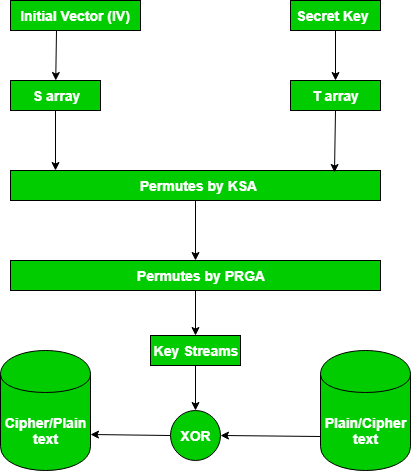GFG App
Open AppBrowser
Continue

# RC4 Encryption Algorithm

RC4 is a stream cipher and variable-length key algorithm. This algorithm encrypts one byte at a time (or larger units at a time). A key input is a pseudorandom bit generator that produces a stream 8-bit number that is unpredictable without knowledge of input key, The output of the generator is called key-stream, is combined one byte at a time with the plaintext stream cipher using X-OR operation.

Example:

```RC4 Encryption
10011000 ? 01010000 = 11001000

RC4 Decryption
11001000 ? 01010000 = 10011000```

Key-Generation Algorithm – A variable-length key from 1 to 256 bytes is used to initialize a 256-byte state vector S, with elements S to S. For encryption and decryption, a byte k is generated from S by selecting one of the 255 entries in a systematic fashion, then the entries in S are permuted again.

Key-Scheduling Algorithm: Initialization: The entries of S are set equal to the values from 0 to 255 in ascending order, a temporary vector T, is created. If the length of the key k is 256 bytes, then k is assigned to T. Otherwise, for a key with length(k-len) bytes, the first k-len elements of T as copied from K, and then K is repeated as many times as necessary to fill T. The idea is illustrated as follow:

## Java

 `for` `    ``i = ``0` `to ``255` `do` `S[i] = i;` `T[i] = K[i mod k - len];`

we use T to produce the initial permutation of S. Starting with S to S, and for each S[i] algorithm swap it with another byte in S according to a scheme dictated by T[i], but S will still contain values from 0 to 255 :

## C++

 `int` `j = 0;` `for` `(``int` `i = 0; i <= 255; i++) {` `    ``j = (j + S[i] + T[i]) % 256;` `    ``swap(S[i], S[j]); ``// Swap S[i] and S[j]` `}`

## Java

 `j = ``0``;` `for` `    ``i = ``0` `to ``255` `do` `    ``{` `        ``j = (j + S[i] + T[i])mod ``256``;` `        ``Swap(S[i], S[j]);` `    ``}`

## Python3

 `j ``=` `0` `for` `i ``in` `range``(``256``):` `    ``j ``=` `(j ``+` `S[i] ``+` `T[i]) ``%` `256` `    ``S[i], S[j] ``=` `S[j], S[i]  ``# Swap S[i] and S[j]`

## C#

 `for` `(``int` `i = 0; i < 256; i++) {` `j = (j + S[i] + T[i]) % 256;` `int` `temp = S[i];` `S[i] = S[j];` `S[j] = temp;` `}`

## Javascript

 `let j = 0;` `for` `(let i = 0; i <= 255; i++) {` `    ``j = (j + S[i] + T[i]) % 256;` `    ``[S[i], S[j]] = [S[j], S[i]]; ``// Swap S[i] and S[j]` `}`

Pseudo random generation algorithm (Stream Generation): Once the vector S is initialized, the input key will not be used. In this step, for each S[i] algorithm swap it with another byte in S according to a scheme dictated by the current configuration of S. After reaching S the process continues, starting from S again

## Java

 `i, j = ``0``;` `while` `(``true``)` `    ``i = (i + ``1``)mod ``256``;` `j = (j + S[i])mod ``256``;` `Swap(S[i], S[j]);` `t = (S[i] + S[j])mod ``256``;` `k = S[t];`

Encrypt using X-Or():News: In September 2015, Microsoft announced the end of using RC4 in Microsoft edge and internet explorer 11. This video gives a clear example of RC4 algorithm.

### Features of the RC4 encryption algorithm:

1. Symmetric key algorithm: RC4 is a symmetric key encryption algorithm, which means that the same key is used for encryption and decryption.
2. Stream cipher algorithm: RC4 is a stream cipher algorithm, which means that it encrypts and decrypts data one byte at a time. It generates a key stream of pseudorandom bits that are XORed with the plaintext to produce the ciphertext.
3. Variable key size: RC4 supports variable key sizes, from 40 bits to 2048 bits, making it flexible for different security requirements.
4. Fast and efficient: RC4 is a fast and efficient encryption algorithm that is suitable for low-power devices and applications that require high-speed data transmission.
5. Widely used: RC4 has been widely used in various applications, including wireless networks, secure sockets layer (SSL), virtual private networks (VPN), and file encryption.
6. Vulnerabilities: RC4 has several vulnerabilities, including a bias in the first few bytes of the keystream, which can be exploited to recover the key. As a result, RC4 is no longer recommended for use in new applications.

1. Fast and efficient: RC4 is a very fast and efficient encryption algorithm, which makes it suitable for use in applications where speed and efficiency are critical.
2. Simple to implement: RC4 is a relatively simple algorithm to implement, which means that it can be easily implemented in software or hardware.
3. Variable key size: RC4 supports variable key sizes, which makes it flexible and adaptable for different security requirements.
4. Widely used: RC4 has been widely used in various applications, including wireless networks, secure sockets layer (SSL), virtual private networks (VPN), and file encryption.